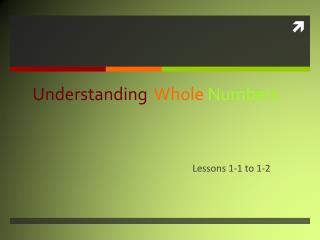# Understanding Whole Numbers - PowerPoint PPT PresentationDownload PresentationUnderstanding Whole Numbers

Understanding Whole Numbers
Download Presentation## Understanding Whole Numbers

- - - - - - - - - - - - - - - - - - - - - - - - - - - E N D - - - - - - - - - - - - - - - - - - - - - - - - - - -
##### Presentation Transcript

1. UnderstandingWhole Numbers Lessons 1-1 to 1-2

2. Vocabulary Forms standard form – a number is written using digits and place value (the regular way to write numbers). (Example: 53,482) expanded form– a number is written as a sum using the place and value of each digit. (Example: fifty thousand + three thousand + four hundred + eighty + two) word form – a number is written in words as you would read it in standard form. (Example: fifty-three thousand, four hundred eighty-two)

3. Place Value Chart

4. How To Read a Large Number • Numbers are grouped in sets of three (each set is called a period). • Only read threenumbers at a time. • Say the name of the period that the numbers are in. • Say “and” for the decimal, but do not say “and” if there isn’t a decimal.

5. Example 4,658,089 Millions period Thousands period Ones period Four million, six hundred fifty-eight thousand, eighty-nine.

6. Whole Number Families Bills family Mills family Thous family Period family

7. Number Lines Numbers towards the right on a number line are larger. As you move to the left on a number line, the numbers get smaller. What’s Bigger? 1 or -2? 1 is larger because it is to the right of the -2. What numbers are smaller than -2? -3 and -4 are both smaller than -2 because they are to the left of -2.

8. Comparing Numbers • Line the numbers up vertically (up and down) by the ones place. • Start at the left and compare the digits. • Move towards the right until you find a difference.

9. Just a Reminder… < means “less than.” (Hint: the point points to the small number) > means “greater than.” (Hint: the open side points to the larger number) =means “equal to.”

10. Example < 45,312 45,321 45,312 45,321 1 is less than 2

11. Example 2 – Put the numbers in order from least to greatest. 321; 345; 354; 29; 1,013; 312; 332 1.2.3.4.5.6.7. 29 smallest largest 321 312 345 321 29 332 354 345 1013 312 354 332 1,013

12. Reading andWritingDecimals “AND” “ths” Lesson 1-2

13. Reading Decimals • Read the number before the decimal point. • Say “AND” when you get to the decimal. • Read the number after the decimal. • Say the name of the place that the decimal ends in.

14. Place Value With Decimals

15. Example 8,243.67 Eight thousand, two hundred forty-three and sixty-seven hundredths Try This: 9,532.41 480. 123 37.06

16. Expanded Form • Write the number that appears before the decimal. • For decimals, place a zero in the ones place. • Also, substitute zeroes for all spaces after the decimal point that come before the digit that you are working with.

17. Example: Write 13.361 in expanded form. + 10 + 3 Remember, deal with the number before the decimal first. 0.3 + 0.06 + 0.001 Then, do the decimal part. Try This: Write 429.715 in expanded form. Answer: 400 + 20 + 9 . 7 + 01 + .005

18. From Words to Standard Form • Read everything that comes before the word “and.” Write that number down. • Place a decimal point at the word “and.” • Read the last word of the sentence to see how many decimal places you need. • Fill in the decimal places with your number. Fill in as far to the right as possible, and use zeroes to fill any empty spaces.

19. Example: Write the following number in standard form:Two hundred six and fifty-four ten-thousandths 5 4 206 . __ __ __ __ 0 0 The word “ten-thousandths” indicates that we need four decimal places. When we clean it up, the answer is 206.0054

20. Classwork • Go to this site that will help you Read and Write Whole Numbers • Whats your Name? • Try this site to read and write Decimals: • Rags To Riches

21. HOMEWORK • Commonly Misspelled Numbers: • hundred • thousand • eight • forty • ninety • Homework: Textbook pg. 10-12, #2-40 Evens • Bonus 2pts: Stretch Your Thinking